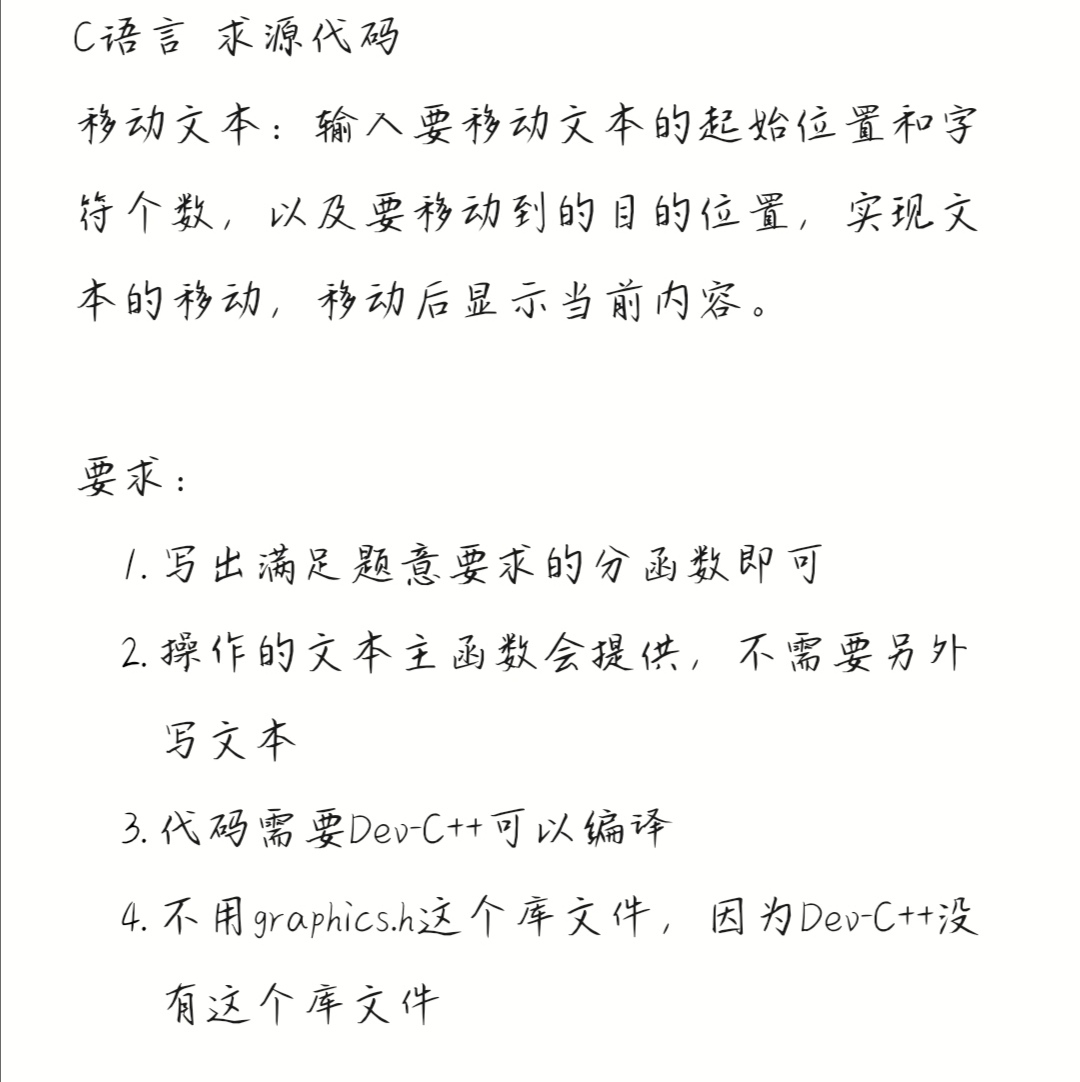2021-06-14 21:45

# 一道C语言编程题，求解答，谢谢！• 点赞
• 写回答
• 关注问题
• 收藏
• 邀请回答

#### 4条回答默认 最新

•已采纳
``````#include<stdio.h>
#include <string.h>
//将s1串中起始位置n，长度l的子串移动到位置m处
char * movetext(char *s1,int n,int l,int m)
{
int len = 0;
int i,j;
for(i=0;s1[i] != 0;i++) //计算字符串s1的长度
len++;
char *p = new char[l];
memcpy(p,s1+n,l); //存储子串
for(i=0;i<len-n-l;i++)  //子串后的字符全部前移l个位置
*(s1+n+i) = *(s1+n+l+i);
len -= l;  //s1删除子串后的长度
if(m >= len-1) //如果插入位置在s1的尾部，则直接合并即可
{
for(i=0;i<l;i++)
*(s1+len+i) = *(p+i);
}
else //插入位置之后的字符后移l个位置
{
for(i=len-1;i>=m;i--)
*(s1+i+l) = *(s1+i);
for(i=0;i<l;i++)
*(s1+m+i) = *(p+i);
}
delete []p;
return s1;
}

int main()
{
char s1[] = "hello world,I love you!";
char *p = movetext(s1,2,2,3);
printf("%s",p);
return 0;
}``````
点赞 评论
•``````#include <stdio.h>
#include <stdlib.h>
#include <graphics.h>

void move();
int main(){
move();
return 0;
}

void move(){
char str = "Hello World!";
int x1=10,x2=600,count;
char ch1,ch2,ch3;

//移动文本：输入要移动文本的起始位置和字符个数，以及要移动到的目的位置，实现文本的移动，移动后显示当前内容。
initgraph(800,600,0);
//设置矩形边框颜色
setcolor(EGERGB(255,255,255));
outtextxy(0,0,"请输入3位的起始位置");
ch1 = getch();
ch2 = getch();
ch3 = getch();
x1 = (ch1-48)*100 + (ch2-48)*10 + (ch3-48);
outtextxy(0,20,"请输入3位的结束位置");
ch1 = getch();
ch2 = getch();
ch3 = getch();
x2 = (ch1-48)*100 + (ch2-48)*10 + (ch3-48);
outtextxy(0,40,"请输入2位的字符个数");
ch1 = getch();
ch2 = getch();
count = (ch1-48)*10 + (ch2-48);

//设置文字的字体
setfont(30,30,"宋体") ;
while(x1<x2){
setcolor(EGERGB(255,255,255));
outtextxy(x1+=10,300,str);
delay_ms(100);
setcolor(EGERGB(0,0,0));
bar(0,300,x2,340);
}
//关闭图形界面
closegraph();

}``````
点赞 评论
•是指输入一段字符串，然后将该字符串中指定位置和长度的子串移动到字符串的另一个位置吗？

点赞 评论
•有问必答小助手 2021-06-17 09:26

您好，我是有问必答小助手，您的问题已经有小伙伴解答了，您看下是否解决，可以追评进行沟通哦~

如果有您比较满意的答案 / 帮您提供解决思路的答案，可以点击【采纳】按钮，给回答的小伙伴一些鼓励哦～～

ps: 问答会员年卡【8折】购 ，限时加赠IT实体书，即可 享受50次 有问必答服务，了解详情>>>https://t.csdnimg.cn/RW5m

点赞 评论